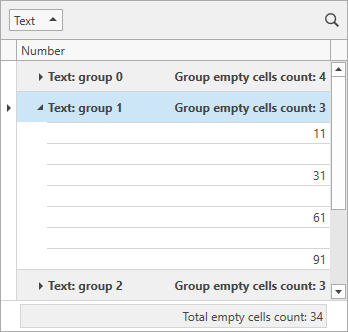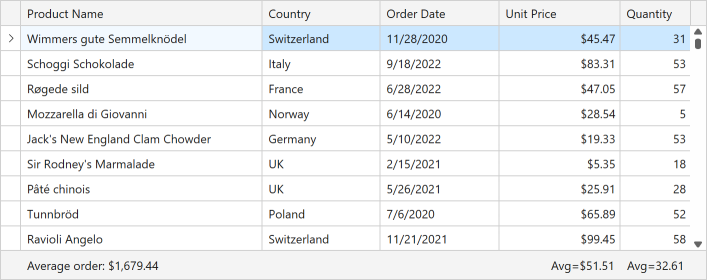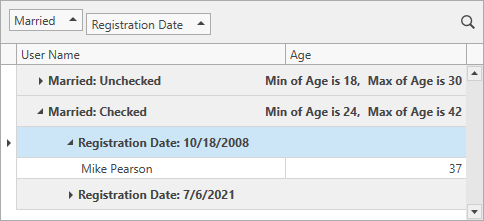# Custom Summary

Total summaries and group summaries contain predefined aggregate functions. These functions allow you to calculate the following:

• The number of data rows (Count).
• The maximum and minimum values (Max and Min).
• The sum and the average value (Sum and Average).

Handle the GridControl.CustomSummary event or use the GridControl.CustomSummaryCommand property to apply custom rules to calculate summaries. Custom summaries allow you to:

• Calculate summaries against records that meet specific criteria.
• Involve multiple data fields in calculations.
• Implement complex summary functions (for example, the standard deviation of a population and so on).

If the GridControl.View property is set to TreeListView, use the TreeListView.CustomSummary event or the TreeListView.CustomSummaryCommand property.

## General Information

To calculate a summary manually:

1. Create a summary item and set its SummaryItemBase.SummaryType property to SummaryItemType.Custom.
2. Create a command that uses a custom algorithm to calculate summary values.
3. Bind this command to the GridControl.CustomSummaryCommand property.

The GridControl calculates its summaries as follows:

Initialization
The GridControl executes the CustomSummary command and sets the SummaryArgs.SummaryProcess property to Start. At this stage, you can initialize summary values (for example, reset internal counters).
Calculation
The GridControl executes the CustomSummary command multiple times, once for each data row in a View or group. The SummaryArgs.SummaryProcess property is set to Calculate. At this stage, you can calculate summaries.
Finalization
The GridControl executes the CustomSummary command and sets the SummaryArgs.SummaryProcess property to Finalize. At this stage, you can assign the calculated summary to the SummaryArgs.TotalValue property.

To skip the Calculation stage and calculate a custom summary at the Initialization or Finalization stage, set the SummaryArgs.TotalValueReady property to true at the Initialization stage. This skips the Calculation stage and starts the Finalization stage.

## Calculate Custom Summaries

The following code sample calculates the total number of empty cells in the specified column:``````<dxg:GridControl ItemsSource="{Binding Items}"
CustomSummaryCommand="{Binding CustomSummaryCommand}">
<dxg:GridControl.Columns>
<dxg:GridColumn FieldName="Text" GroupIndex="0" />
<dxg:GridColumn FieldName="Number" />
</dxg:GridControl.Columns>
<dxg:GridControl.View>
<dxg:TableView AutoWidth="True"
TotalSummaryPosition="Bottom" />
</dxg:GridControl.View>
<dxg:GridControl.TotalSummary>
<dxg:GridSummaryItem DisplayFormat="Total empty cells count: {0}"
FieldName="Number"
SummaryType="Custom" />
</dxg:GridControl.TotalSummary>
<dxg:GridControl.GroupSummary>
<dxg:GridSummaryItem DisplayFormat="Group empty cells count: {0}"
FieldName="Number"
SummaryType="Custom" />
</dxg:GridControl.GroupSummary>
</dxg:GridControl>
``````
``````using DevExpress.Mvvm;
using DevExpress.Mvvm.DataAnnotations;
using DevExpress.Mvvm.Xpf;
// ...
public class MainViewModel : ViewModelBase {
// ...
[Command]
public void CustomSummary(RowSummaryArgs args) {
if(args.SummaryItem.PropertyName != "Number")
return;
if(args.SummaryProcess == SummaryProcess.Start) {
args.TotalValue = 0;
}
if(args.SummaryProcess == SummaryProcess.Calculate) {
if(IsEmptyCell(args.FieldValue))
args.TotalValue = (int)args.TotalValue + 1;
}
}
bool IsEmptyCell(object fieldValue) {
return !((int?)fieldValue).HasValue;
}
}
``````

## Calculate Custom Summaries Based on Predefined Summaries

The GridControl calculates custom summaries after predefined summaries (Count, Sum, Min, and so on). As a result, you can use predefined summary values to calculate custom summaries:

1. Create a custom summary.
2. Handle the GridControl.CustomSummary / TreeListView.CustomSummary event.
3. At the Initialization stage, set the e.TotalValueReady property to `true` to skip the Calculation stage.
4. Use the DataControlBase.GetTotalSummaryValue method to obtain predefined summaries at the Finalization stage.``````<dxg:GridControl ...
CustomSummary="grid_CustomSummary">
<dxg:GridColumn FieldName="ProductName"/>
<dxg:GridColumn FieldName="UnitPrice"/>
<dxg:GridColumn FieldName="Quantity"/>
<dxg:GridControl.TotalSummary>
<dxg:GridSummaryItem x:Name="avgPrice" FieldName="UnitPrice" SummaryType="Average"/>
<dxg:GridSummaryItem x:Name="avgQuantity" FieldName="Quantity" SummaryType="Average"/>
<dxg:GridSummaryItem ShowInColumn="ProductName" SummaryType="Custom"
DisplayFormat="{}Average order: {0:c}"/>
</dxg:GridControl.TotalSummary>
<dxg:GridControl.View>
<dxg:TableView ...
TotalSummaryPosition="Bottom">
</dxg:TableView>
</dxg:GridControl.View>
</dxg:GridControl>
``````
``````private void grid_CustomSummary(object sender, DevExpress.Data.CustomSummaryEventArgs e) {
if (e.IsTotalSummary) {
switch (e.SummaryProcess) {
case DevExpress.Data.CustomSummaryProcess.Start:
break;
case DevExpress.Data.CustomSummaryProcess.Finalize:
var averagePrice = (decimal)grid.GetTotalSummaryValue(avgPrice);
var averageQuantity = (decimal)grid.GetTotalSummaryValue(avgQuantity);
e.TotalValue = averagePrice * averageQuantity;
break;
}
}
}
``````

You can use the e.GetGroupSummary method to obtain predefined group summary values.

## Specify Whether to Calculate Summaries

The CustomSummaryExists event or the CustomSummaryExistsCommand property allows you to specify which summaries should be calculated and displayed.

The following example calculates group summaries only for the top group level:``````<dxg:GridControl x:Name="grid"
ItemsSource="{Binding AccountList}"
CustomSummaryExistsCommand="{Binding CustomSummaryExistsCommand}">
<!-- ... -->
<dxg:GridControl.GroupSummary>
<dxg:GridSummaryItem FieldName="Age" SummaryType="Min"/>
<dxg:GridSummaryItem FieldName="Age" SummaryType="Max"/>
</dxg:GridControl.GroupSummary>
</dxg:GridControl>
``````
``````using DevExpress.Mvvm;
using DevExpress.Mvvm.DataAnnotations;
using DevExpress.Mvvm.Xpf;
// ...
public class MainViewModel : ViewModelBase {
// ...
[Command]
public void CustomSummaryExistsCommand(RowSummaryExistsArgs args) {
args.Exists = args.GroupPath.GroupLevel == 0;
}
}
``````